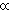HOME MATH DICTIONARY DOWNLOAD FEEDBACK DISCLAIMER
 Question: What do you mean by Inverse Proportion ? Answer: When the average speed for a journey of 100 km is doubled, the time taken is halved. The time taken, t hours is said to be inversely proportional to the speed u km per hour. As u increases, t decreases according to the algebraic relationship t = 100 / u In general when y is inversely proportional to x there is an algebraic relationship between x and y of the form y = a / x Where a is a constant. y is inversely proportional to x is often written y1 / x .